# Organize data divided by folder with Python

## In 3 lines

• Get the `glob.glob` folder list.
• Execute processing for each folder (if necessary) `subprocess.Popen`
• Use `pandas` to combine the output text files for each folder into` DataFrame` and organize the data.

## Get a list of folder names

### Standard version (OK in standard environment)

``````import os
dir = [d for d in os.listdir(".") if os.path.isdir(d)]
``````

### A cooler way (regular expressions can be used)

#### `Windows`

``````
import glob
dir = glob.glob(os.path.join("*",""))
``````

#### `Mac`

``````
dir = glob.glob("*/")
``````

#### Regular expression usage example

Example of searching for folders case01, case02, ...

``````dir = glob.glob(os.path.join("case*",""))
``````

If you want to get only a text file (.txt).

``````dir = glob.glob("*.txt")
``````

## Execute the processing program for each folder

``````import shutil
import subprocess

for f in dir:

# copy files from local folder to target folder
for fi in cp_files:
shutil.copy(fi,f)

# remove files at target folder
rm_files=['y.out','out.tsv']
for fi in rm_files:
if os.path.exists(os.path.join(f,fi)):
os.remove(os.path.join(f,fi))

``````

## Process text data organized by folder with pandas

The data is in tab format (.tsv), and the index column and data column are assumed from the left.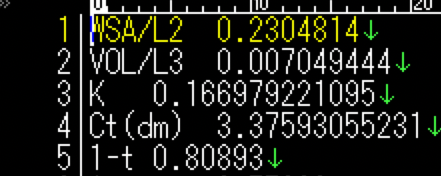Data reading may be handled by try: because the above processing program may fail. The error folder needs to be output. It is convenient to prepare the index by processing from the folder name later.

``````import pandas as pd

dfs=pd.DataFrame()

for f in dir:
# case01\\ => case01
index_name = os.path.split(f)

# Error handle
try:
# Data structure {col.0 : index, col.1 : Data}
dfs[index_name]=df.iloc[:,0]
except:
print("Error in {0}".foramt(index_name))

# make index
dfs.index = df.index
``````

Let's check the data. (Why is there a "0" line, but I don't care because it will disappear later)

``````dfs.head()
``````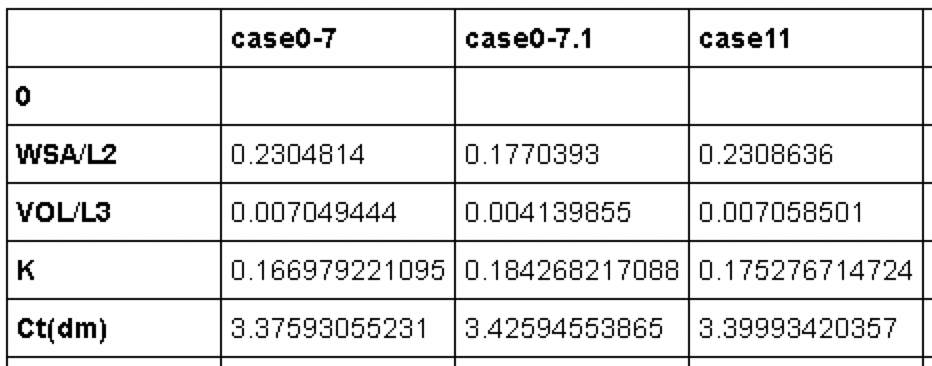## Something done with pandas

First, it's easier to handle if you swap the rows and columns.

``````dfsT = dfs.T
``````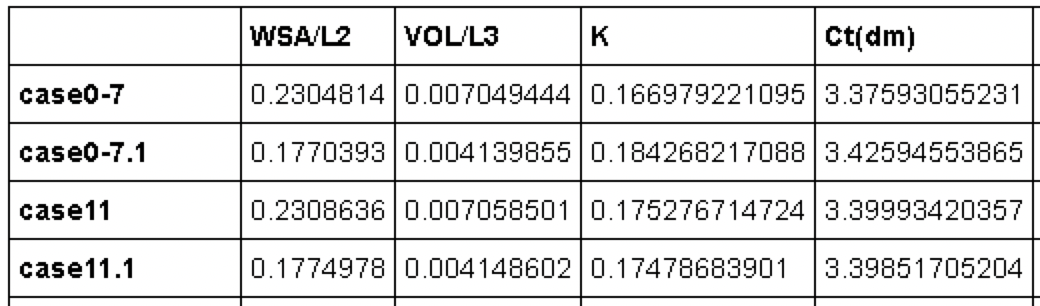First, processing of missing data (NaN).

``````dfsT = dfsT.dropna()
``````

Appropriately from here.

For example, use a fancy index to process conditional data. (Here, an example in which the WSA / L2 column outputs data of 0.2 or more)

``````dfsT_select = dfsT[dfsT["WSA/L2"] > 0.2]
``````

### Visualization with matplotlib

``````import matplotlib.pyplot as plt

plt.bar(range(len(dfsT)),dfsT["WSA/L2"], \
tick_label=dfsT.index)
plt.show()
``````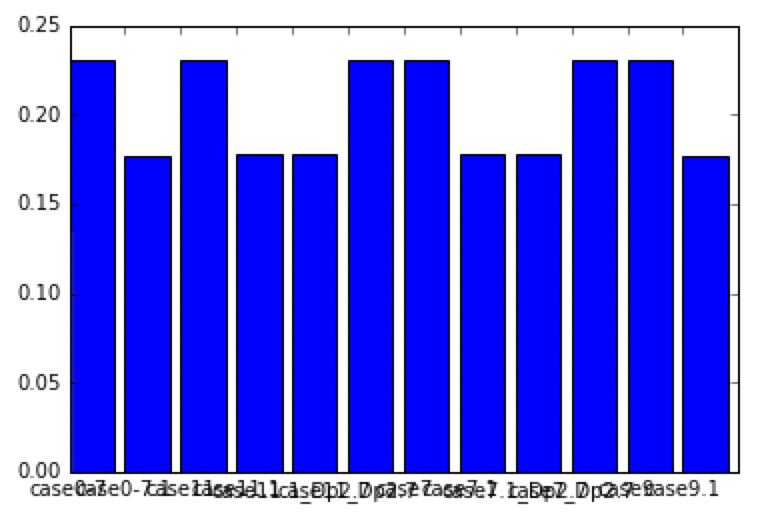Adjustment of horizontal axis

``````fig, ax = plt.subplots()
ax.bar(range(len(dfsT)),dfsT["WSA/L2"], \
tick_label=dfsT.index)
labels = ax.get_xticklabels()
plt.setp(labels, rotation=45, fontsize=10);
``````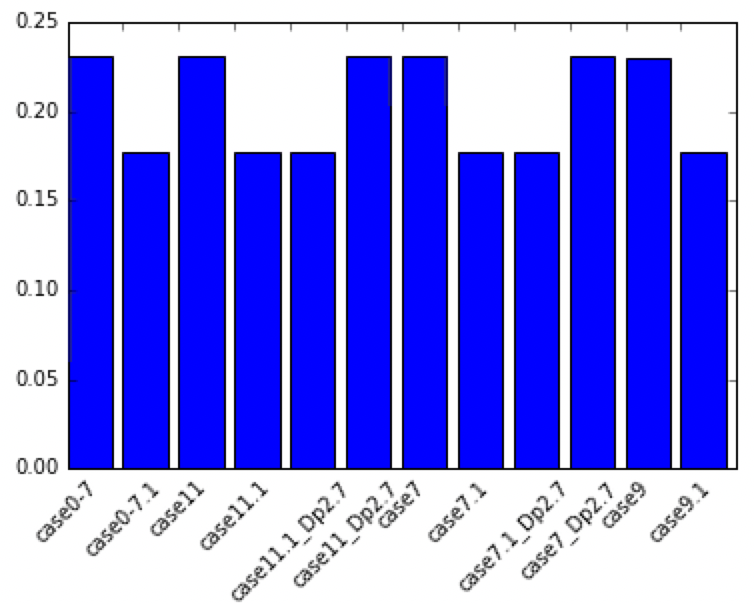## Utilization in Excel (output)

Many people ask me to use Excel for the data, so I'll give it to you.

``````dfs.to_excel("addup.xlsx")
``````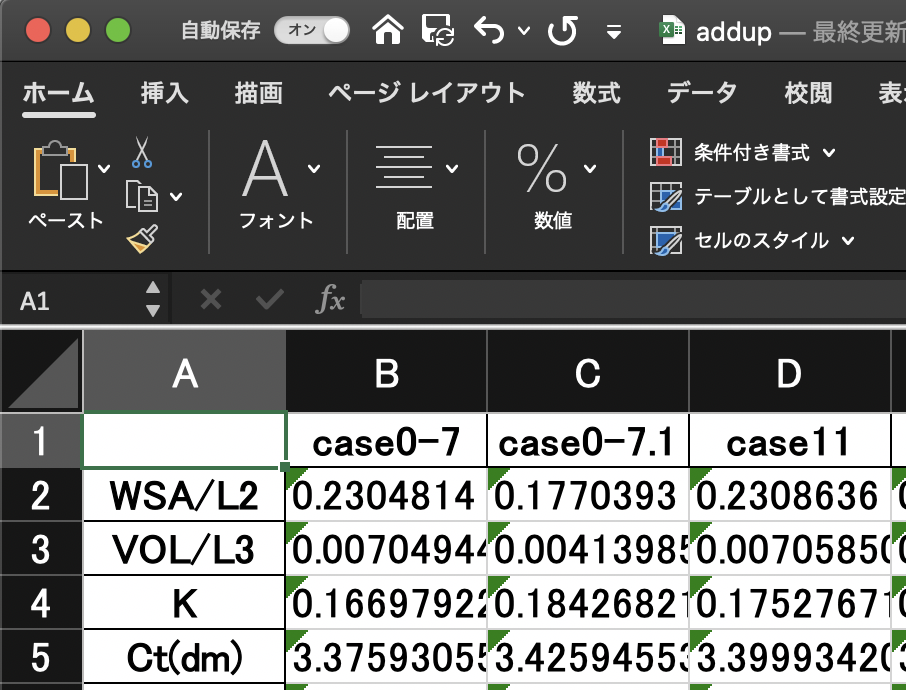If the text format is acceptable, for example:

``````dfs.to_csv("addup.tsv",sep='\t')
``````